# What is the meaning of trigonometric functions

## Trigonometric functions¶

### The Arcus functions¶

The Arcus functions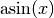,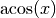and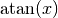give at a given value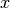the associated angle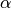at; they are thus the inverse functions of the trigonometric functions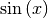,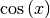and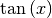. For example isthe angle in the unit circle whose sine is equalis.

Since the sine, cosine and tangent functions are not bijective due to their periodicity, their range of definition must be restricted when creating the respective inverse function. The arc functions are usually defined with the following domains:

The value ranges of the arc functions agree with the above definition ranges of the original trigonometric functions.

Remarks: